Main Content

# sbiompgsa

Perform multiparametric global sensitivity analysis (requires Statistics and Machine Learning Toolbox)

## Syntax

``mpgsaResults = sbiompgsa(modelObj,params,classifiers)``
``mpgsaResults = sbiompgsa(modelObj,samples,classifiers)``
``mpgsaResults = sbiompgsa(simdata,samples,classifiers)``
``mpgsaResults = sbiompgsa(___,Name,Value)``

## Description

example

````mpgsaResults = sbiompgsa(modelObj,params,classifiers)` performs a multiparametric global sensitivity analysis (MPGSA)  of `classifiers` with respect to model parameters `params` on a SimBiology model `modelObj`. `params` are model quantities (sensitivity inputs) and `classifiers` define the expressions of model responses (model outputs).```
````mpgsaResults = sbiompgsa(modelObj,samples,classifiers)` uses parameter `samples` to perform a multiparametric global sensitivity analysis of `classifiers`.```

example

````mpgsaResults = sbiompgsa(simdata,samples,classifiers)` uses model simulation data `simdata` to perform a multiparametric global sensitivity analysis of `classifiers`.```

example

````mpgsaResults = sbiompgsa(___,Name,Value)` uses additional options specified by one or more name-value pair arguments. Available name-value pairs differ depending on which syntax you are using.```

## Examples

collapse all

`sbioloadproject tmdd_with_TO.sbproj`

Get the active configset and set the target occupancy (`TO`) as the response.

```cs = getconfigset(m1); cs.RuntimeOptions.StatesToLog = 'TO';```

Simulate the model and plot the `TO` profile.

`sbioplot(sbiosimulate(m1,cs));`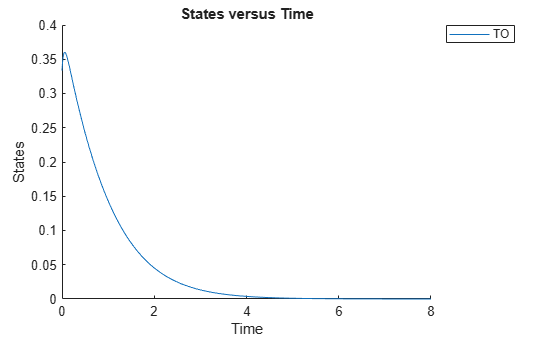Define an exposure (area under the curve of the TO profile) threshold for the target occupancy.

`classifier = 'trapz(time,TO) <= 0.1';`

Perform MPGSA to find sensitive parameters with respect to the TO. Vary the parameter values between predefined bounds to generate 10,000 parameter samples.

```% Suppress an information warning that is issued during simulation. warnSettings = warning('off', 'SimBiology:sbservices:SB_DIMANALYSISNOTDONE_MATLABFCN_UCON'); rng(0,'twister'); % For reproducibility params = {'kel','ksyn','kdeg','km'}; bounds = [0.1, 1; 0.1, 1; 0.1, 1; 0.1, 1]; mpgsaResults = sbiompgsa(m1,params,classifier,'Bounds',bounds,'NumberSamples',10000)```
```mpgsaResults = MPGSA with properties: Classifiers: {'trapz(time,TO) <= 0.1'} KolmogorovSmirnovStatistics: [4x1 table] ECDFData: {4x4 cell} SignificanceLevel: 0.0500 PValues: [4x1 table] SupportHypothesis: [10000x1 table] Observables: {'TO'} ParameterSamples: [10000x4 table] SimulationInfo: [1x1 struct] ```

Plot the quantiles of the simulated model response.

`plotData(mpgsaResults);`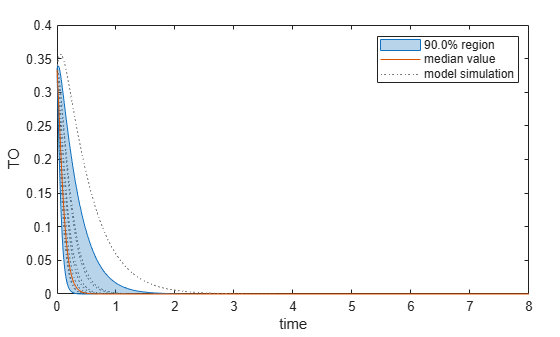Plot the empirical cumulative distribution functions (eCDFs) of the accepted and rejected samples. Except for `km`, none of the parameters shows a significant difference in the eCDFs for the accepted and rejected samples. The `km` plot shows a large Kolmogorov-Smirnov (K-S) distance between the eCDFs of the accepted and rejected samples. The K-S distance is the maximum absolute distance between two eCDFs curves.

```h = plot(mpgsaResults); % Resize the figure. pos = h.Position(:); h.Position(:) = [pos(1) pos(2) pos(3)*2 pos(4)*2];```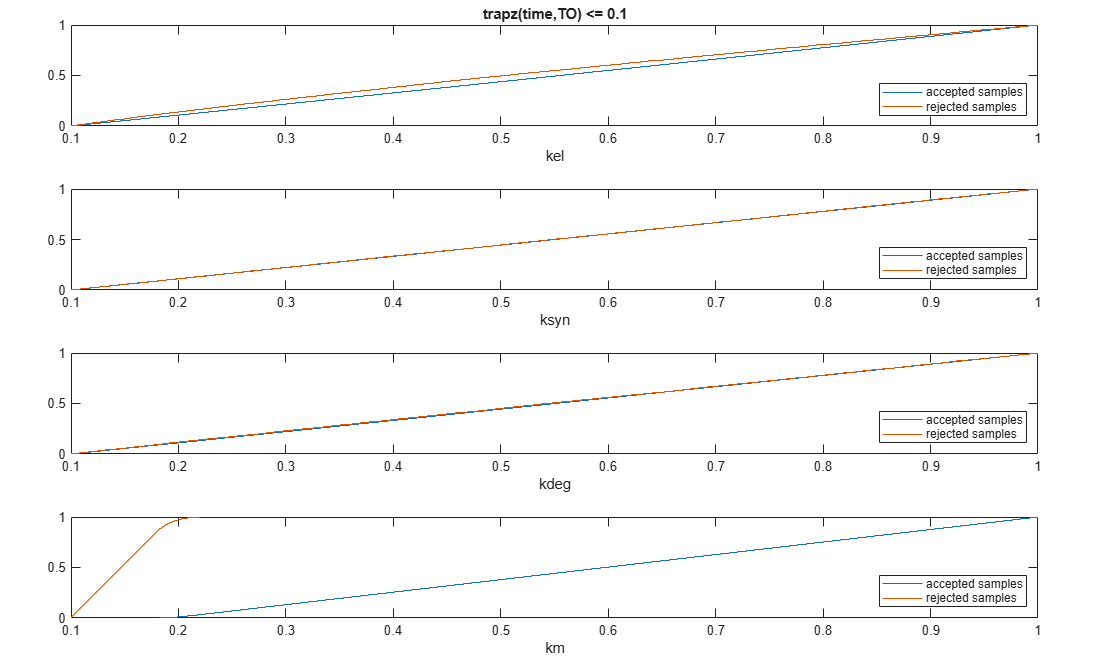To compute the K-S distance between the two eCDFs, SimBiology uses a two-sided test based on the null hypothesis that the two distributions of accepted and rejected samples are equal. See `kstest2` (Statistics and Machine Learning Toolbox) for details. If the K-S distance is large, then the two distributions are different, meaning that the classification of the samples is sensitive to variations in the input parameter. On the other hand, if the K-S distance is small, then variations in the input parameter do not affect the classification of samples. The results suggest that the classification is insensitive to the input parameter. To assess the significance of the K-S statistic rejecting the null-hypothesis, you can examine the p-values.

`bar(mpgsaResults)`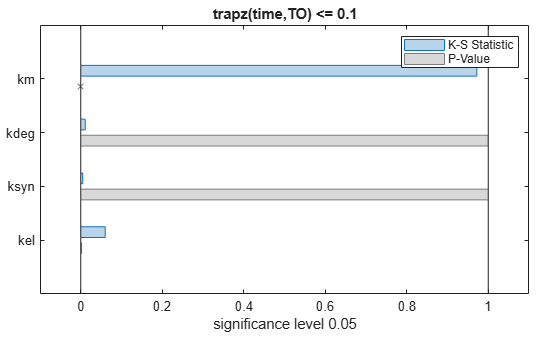The bar plot shows two bars for each parameter: one for the K-S distance (K-S statistic) and another for the corresponding p-value. You reject the null hypothesis if the p-value is less than the significance level. A cross (`x`) is shown for any p-value that is almost 0. You can see the exact p-value corresponding to each parameter.

`[mpgsaResults.ParameterSamples.Properties.VariableNames',mpgsaResults.PValues]`
```ans=4×2 table Var1 trapz(time,TO) <= 0.1 ________ _____________________ {'kel' } 0.0021877 {'ksyn'} 1 {'kdeg'} 0.99983 {'km' } 0 ```

The p-values of `km` and `kel` are less than the significance level (0.05), supporting the alternative hypothesis that the accepted and rejected samples come from different distributions. In other words, the classification of the samples is sensitive to `km` and `kel` but not to other parameters (`kdeg` and `ksyn`).

You can also plot the histograms of accepted and rejected samples. The historgrams let you see trends in the accepted and rejected samples. In this example, the histogram of `km` shows that there are more accepted samples for larger `km` values, while the `kel` histogram shows that there are fewer rejected samples as `kel` increases.

```h2 = histogram(mpgsaResults); % Resize the figure. pos = h2.Position(:); h2.Position(:) = [pos(1) pos(2) pos(3)*2 pos(4)*2];```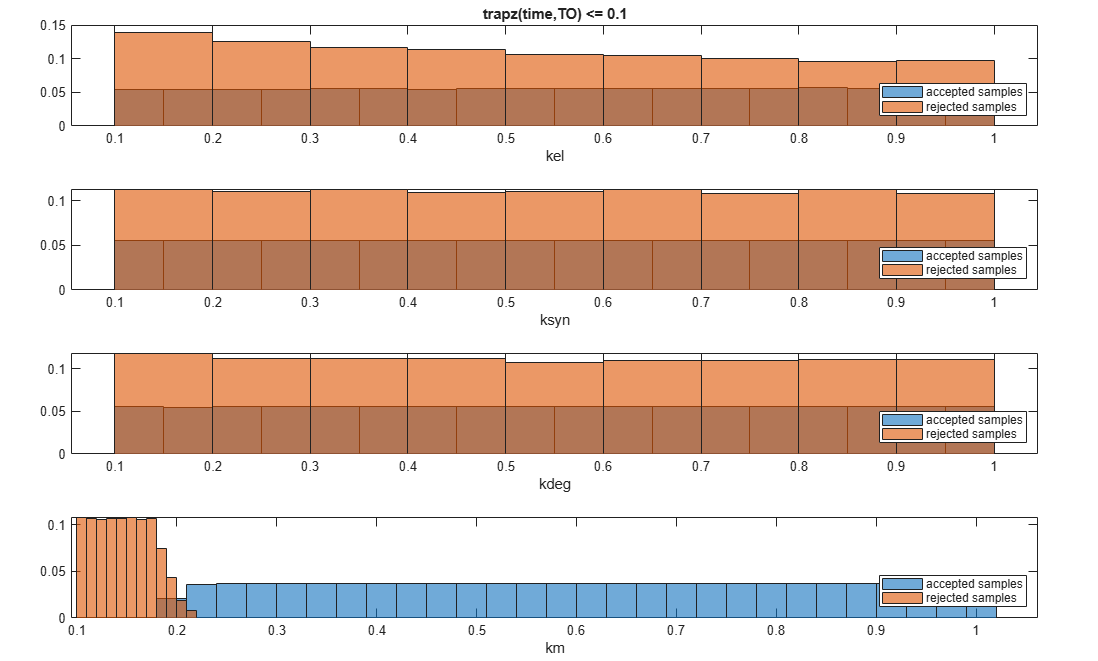Restore the warning settings.

`warning(warnSettings);`

## Input Arguments

collapse all

SimBiology model, specified as a SimBiology `model object`.

Names of model parameters, species, or compartments, specified as a character vector, string, string vector, or cell array of character vectors.

Example: `["k1","k2"]`

Data Types: `char` | `string` | `cell`

Model simulation data, specified as a vector of `SimData` objects.

Expressions of model responses, specified as a character vector, string, string vector, or cell array of character vectors. Specify expressions referencing simulated model quantities, observables, time, or MATLAB functions that are on the path.

Each classifier must evaluate to a logical vector of the same length as the number of parameter samples. Entities, such as model quantities or observables, referenced in a classifier expression are evaluated as a matrix with columns containing time courses of the simulated quantity values. Each column represents one sample. For details, see Multiparametric Global Sensitivity Analysis (MPGSA).

If you specify a `SimData` object as the first input, each quantity or `observable` referenced by the classifier must resolve to a logged component in the `SimData` object.

Example: ```"max(Central.Drug(time > 1,:),[],1) <= 7"```

Data Types: `char` | `string` | `cell`

Sampled values of model quantities, specified as a table. The variable names of the table indicate the quantity names.

Data Types: `table`

### Name-Value Pair Arguments

Example: ```mpgsaResults = sbiompgsa(m1,{'k1','k2'},classifier,'Bounds',[0.5 5;0.1 1])``` specifies parameter bounds for k1 and k2.

Specify one or more comma-separated pairs of `Name,Value` arguments. `Name` is the argument name and `Value` is the corresponding value. `Name` must appear inside quotes. You can specify several name and value pair arguments in any order as `Name1,Value1,...,NameN,ValueN`.

Note

Depending on which syntax you use, available name-value pairs differ.

For the First Syntax

collapse all

Parameter bounds, specified as the comma-separated pair consisting of `'Bounds'` and a numeric matrix with two columns. The first column contains the lower bounds and the second column contains the upper bounds. The number of rows must be equal to the number of parameters in `params`.

If a parameter has a nonzero value, the default bounds are ±10% of the value. If the parameter value is zero, the default bounds are `[0 1]`.

Example: `[0.5 5]`

Data Types: `double`

Number of samples drawn to perform multiparametric global sensitivity analysis, specified as the comma-separated pair consisting of `'NumberSamples'` and a positive integer.

Data Types: `double`

Method to generate parameter samples, specified as the comma-separated pair consisting of `'SamplingMethod'` and a character vector or string. Valid options are:

• `'Sobol'` — Use the low-discrepancy Sobol sequence to generate samples.

• `'Halton'` — Use the low-discrepancy Halton sequence to generate samples.

• `'lhs'` — Use the low-discrepancy Latin hypercube samples.

• `'random'` — Use uniformly distributed random samples.

Data Types: `char` | `string`

For the First and Second Syntaxes

collapse all

Doses to use during model simulations, specified as the comma-separated pair consisting of `'Doses'` and a `ScheduleDose` or `RepeatDose` object or a vector of dose objects.

Variants to apply before model simulations, specified as the comma-separated pair consisting of `'Variants'` and a variant object or vector of variant objects.

When there are multiple variants and if there are duplicate specifications for a property's value, the last occurrence for the property value in the array of variants is used during simulation.

Simulation stop time, specified as the comma-separated pair consisting of `'StopTime'` and a nonnegative scalar. If you specify neither `StopTime` nor `OutputTimes`, the function uses the stop time from the active configuration set of the model. You cannot specify both `StopTime` and `OutputTimes`.

Data Types: `double`

Flag to run model simulations in parallel, specified as the comma-separated pair consisting of `'UseParallel'` and `true` or `false`.

Data Types: `logical`

Flag to turn on model acceleration, specified as the comma-separated pair consisting of `'Accelerate'` and `true` or `false`.

Data Types: `logical`

For All Syntaxes

collapse all

Simulation output times, specified as the comma-separated pair consisting of `'OutputTimes'` and a numeric vector. The function computes model responses at these output time points. You cannot specify both `StopTime` and `OutputTimes`. By default, the function uses the output times of the first model simulation.

Example: `[0 1 2 3.5 4 5 5.5]`

Data Types: `double`

Significance level for Kolmogorov-Smirnov test, specified as the comma-separated pair consisting of `'SignificanceLevel'` and a numeric scalar between 0 and 1. For details, see Two-Sample Kolmogorov-Smirnov Test (Statistics and Machine Learning Toolbox).

Example: `0.1`

Data Types: `double`

Method for interpolation of model simulations to new output times, specified as the comma-separated pair consisting of `'InterpolationMethod'` and a character vector or string. The valid options follow.

• `"interp1q"` — Use the `interp1q` function.

• Use the `interp1` function by specifying one of the following methods:

• `"nearest"`

• `"linear"`

• `"spline"`

• `"pchip"`

• `"v5cubic"`

• `"zoh"` — Specify the zero-order hold.

Data Types: `char` | `string`

## Output Arguments

collapse all

Multiparametric global sensitivity analysis results, returned as a `SimBiology.gsa.MPGSA` object. The object also contains model simulation data used to perform MPGSA.

## More About

collapse all

### Multiparametric Global Sensitivity Analysis (MPGSA)

Multiparametric global sensitivity analysis lets you study the relative importance of parameters with respect to a classifier defined by model responses. A classifier is an expression of model responses that evaluates to a logical vector. `sbiompgsa` implements the MPSA method described by Tiemann et. al. (see supporting information text S2) .

`sbiompgsa` performs the following steps.

1. Generate N parameter samples using a sampling method. `sbiompgsa` stores these samples as a table in a property, `mpgsaResults.ParameterSamples`, of the returned object. The number of rows is equal to the number of samples and the number of columns is equal to the number of input parameters.

Tip

You can specify N and the sampling method using the `'NumberSamples'` and `'SamplingMethod'` name-value pair arguments, respectively, when you call `sbiompgsa`.

2. Calculate the model response by simulating the model for each parameter set, which is a single realization of the model parameter values. In this case, a parameter set is equal to a row in the `ParameterSamples` table.

3. Evaluate the classifier. A classifier is an expression that evaluates to a logical vector. For instance, if your model response is the AUC of plasma drug concentration, you can define a classifier with a toxicity threshold of 0.8 where the AUC of the drug concentration above that threshold is considered toxic.

4. Parameter sets are then separated into two different groups, such as accepted (nontoxic) and rejected (toxic) groups.

5. For each input parameter, compute the empirical cumulative distribution functions (`ecdf` (Statistics and Machine Learning Toolbox)) of accepted and rejected sample values.

6. Compare the two eCDFs of accepted and rejected groups using the Two-Sample Kolmogorov-Smirnov Test (Statistics and Machine Learning Toolbox) to compute the Kolmogorov-Smirnov distance. The default significance level of the Kolmogorov-Smirnov test is `0.05`. If two eCDFs are similar, the distance is small, meaning the model response is not sensitive with respect to the input parameter. If two eCDFs are different, the distance is large, meaning the model response is sensitive to the parameter.

Note

The Kolmogorov-Smirnov test assumes that the samples follow a continuous distribution. Make sure that the eCDF plots are continuous as you increase the number of samples. If eCDFs are not continuous but step-like in the limit of infinite samples, then the results might not reflect the true sensitivities.

 Tiemann, Christian A., Joep Vanlier, Maaike H. Oosterveer, Albert K. Groen, Peter A. J. Hilbers, and Natal A. W. van Riel. “Parameter Trajectory Analysis to Identify Treatment Effects of Pharmacological Interventions.” Edited by Scott Markel. PLoS Computational Biology 9, no. 8 (August 1, 2013): e1003166. https://doi.org/10.1371/journal.pcbi.1003166.

## SupportGet trial now